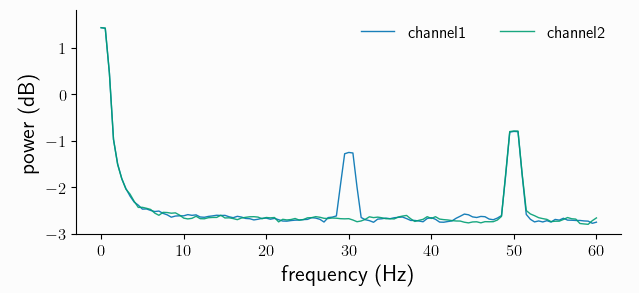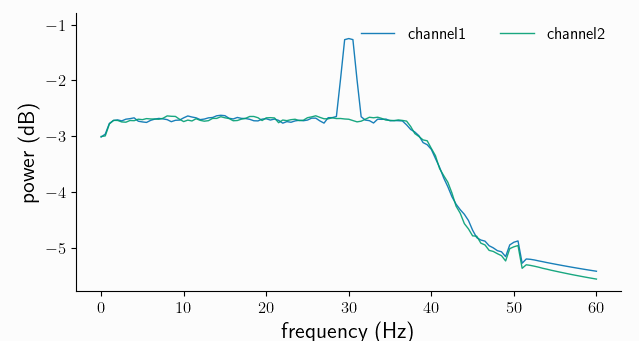# Preprocessing#

Raw data often contains unwanted signal components: offsets, trends or even oscillatory nuisance signals. Syncopy has a dedicated `preprocessing()` function to clean up and/or transform the data.

Let’s start by creating a new synthetic signal with confounding components:

```# built-in synthetic data generators
import syncopy as spy
from syncopy import synthdata as spy_synth

cfg_synth = spy.StructDict()
cfg_synth.nTrials = 150
cfg_synth.samplerate = 500
cfg_synth.nSamples = 1000
cfg_synth.nChannels = 2

# 30Hz undamped harmonig
harm = spy_synth.harmonic(cfg_synth, freq=30)

# a linear trend
lin_trend = spy_synth.linear_trend(cfg_synth, y_max=3)

# a 2nd 'nuisance' harmonic
harm50 = spy_synth.harmonic(cfg_synth, freq=50)

# finally the white noise floor
wn = spy_synth.white_noise(cfg_synth)
```

Here we used a `cfg` dictionary to assemble all needed parameters, a concept we adopted from FieldTrip

## Dataset Arithmetics#

If the shape of different Syncopy objects match exactly (`nSamples`, `nChannels` and `nTrials` are all the same), we can use standard Python arithmetic operators like +, -, * and / directly. Here we want a linear superposition, so we simply add everything together:

```# add noise, trend and the nuisance harmonic
data_nui = harm + wn + lin_trend + harm50
# also works for scalars
data_nui = data_nui + 5
```

If we now do a spectral analysis, the power spectra are confounded by all our new signal components:

```cfg = spy.StructDict()
cfg.tapsmofrq = 1
cfg.foilim = [0, 60]
cfg.polyremoval = None
cfg.keeptrials = False   # trial averaging
fft_nui_spectra = spy.freqanalysis(data_nui, cfg)
```

Note

We explicitly set `polyremoval=None` to see the full effect of our confounding signal components. The default for `freqanalysis()` is `polyremoval=0`, which removes polynoms of 0th order: constant offsets (de-meaning).

Hint

We did not specify the `method` parameter for the `freqanalysis()` call as multi-tapered Fourier analysis (`method='mtmfft'`) is the default. To learn about the defaults of any Python function you can inspect its signature with `spy.freqanalysis?` or `help(spy.freqanalysis)` typed into an interpreter

Let’s see what we got:

```fft_nui_spectra.singlepanelplot()
```We see strong low-frequency components, originating from both the offset and the trend. We also see the nuisance signal spectral peak at 50Hz.

## Filtering#

Filtering of signals in general removes/suppresses unwanted signal components. This can be done both in the time-domain and in the frequency-domain. For offsets and (low-order) polynomial trends, fitting a model directly in the time domain, and subtracting the obtained trend, is the preferred solution. This can be controlled in Syncopy with the `polyremoval` parameter, which is also directly available in `freqanalysis()`.

Removing signal components in the frequency domain is typically done with finite impulse response (FIR) filters or infinite impulse response (IIR) filters. Syncopy supports one of each kind, a FIR windowed sinc and the Butterworth filter from the IIR family. For both filters we have low-pass (`'lp'`), high-pass (`'hp'`), band-pass (`'bp'`) and band-stop(Notch) (`'bp'`) designs available.

To clean up our dataset above, we remove the linear trend and apply a low-pass 12th order Butterworth filter:

```data_pp = spy.preprocessing(data_nui,
filter_class='but',
filter_type='lp',
polyremoval=1,
freq=40,
order=12)
```

Now let’s reuse our `cfg` from above to repeat the spectral analysis with the preprocessed data:

```spec_pp = spy.freqanalysis(data_pp, cfg)
spec_pp.singlepanelplot()
```As expected for a low-pass filter, all frequencies above 40Hz are strongly attenuated (note the log scale, so the suppression is around 2 orders of magnitude). We also removed the low-frequency components from the offset and trend, but acknowledge that we also lost a bit of the original white noise power around 0-2Hz. Importantly, the spectral power of our frequency band of interest, around 30Hz, remained virtually unchanged.